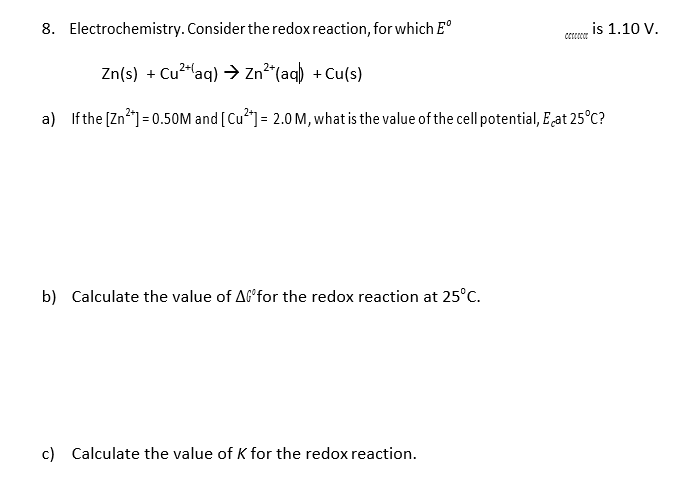Electrochemistry. Consider the redoxreaction, for which E8.Co S 1.10 v.Zn(s) Cuaq) - Zn (aq) Cu(s)Ifthe [Zn21-0.50M and [Cu2]- 2.0M, what is the value ofthe cell potential, E,at 25c?a)Calculate the value of M"for the redox reaction at 25°C.b)Calculate the value of K for the redox reaction.c)

Questionhelp_outlineImage TranscriptioncloseElectrochemistry. Consider the redoxreaction, for which E 8. Co S 1.10 v. Zn(s) Cuaq) - Zn (aq) Cu(s) Ifthe [Zn21-0.50M and [Cu2]- 2.0M, what is the value ofthe cell potential, E,at 25c? a) Calculate the value of M"for the redox reaction at 25°C. b) Calculate the value of K for the redox reaction. c) fullscreen
Step 1

Oxidation state or oxidation number of an atom refers to the charge on that atom that it would have been carried if the compound is composed only of the ions.

Redox reactions are those chemical reactions in which the transfer of electrons takes place among the species undergoing redox reactions. This means that these reactions are accompanied by change in the oxidation number of the molecule or the ions by loss or gain of electrons by that species. If a chemical species gains electrons during a redox chemical reaction then it is said to be reduced while if it loose electrons it is said to be oxidized in the redox reaction.

Step 2

Since every redox reaction is made up of two half reactions where  one is the oxidation half reaction which shows the species that looses electrons  and the other is the reduction half reaction which is used to depict the reaction in which the chemical species gains electrons .

Electrode potential refers to the potential difference which is developed between the electrolyte and the electrode in an electrochemical cell. The term Eo cell refers to the standard state potential that means that its value is determined under standard conditions  which include concentrations of 1M  and a pressure of about 1 atm while the Ecell refers to the electrode potential which is not determined under standard conditions.

Step 3

The equation which shows the relation between these electrode potentials and the concentration of the ...

Want to see the full answer?

See Solution

Want to see this answer and more?

Our solutions are written by experts, many with advanced degrees, and available 24/7

See Solution
Tagged in

Electrochemistry